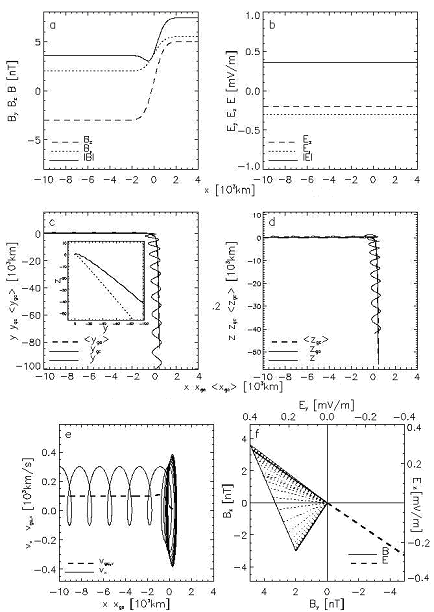Home  Research  Publications  Personnel  Workshops  Intranet English RomanaSpace Plasma Simulations and ModelsImpulsive penetration mechanismInvestigation of non-uniform electromagnetic fields with test-particle simulationsSolutions of the steady-state Vlasov equation by numerical integration of its characteristicsMagnetohydrostatic model of mirror modesInvestigation of non-uniform electromagnetic fields with test-particles simulationsIn the outer space the magnetic field experiences sharp variations of direction and/or amplitude within thin regions called by Burlaga (1969) 'directional discontinuities' (DD). The typical thickness of DDs is of the order of the proton Larmor radii. Thus they are 'kinetic-scale' phenomena and a kinetic treatment is in order (Lemaire and Burlaga, 1976). The 3D surface of the magnetopause is a typical example of a directional discontinuity. We have investigated the magnetic field distribution typical for 'tangential discontinuities' (TD) that are the subclass of DDs that satisfy the condition that the normal component of the magnetic field is zero inside the discontinuity.In-situ observations (e.g. Berchem and Russell, 1982; Papamastorakis et al., 1984) showed examples of sheared distribution of the magnetic field at the dayside magnetopause. In these examples the angle of shear, between the external (magnetosheath) with respect to the internal (magnetospheric) magnetic field took values ranging between 00 and +/-1800. Highly-sheared and antiparallel B-field topology are precisely assigned to southward IMF and considered in the steady-state 2D-reconnection models (Priest and Forbes, 1986). We have numerically computed the orbits of charged particles having energies typical for the solar wind (Echim, 2002). We have also integrated the trajectories of the corresponding guiding centers. The test-particles are injected normal to a TD for which the magnetic field is ''sheared'' and its normal component is equal to zero (Bn=0). This magnetic field distribution would be encountered at the dayside magnetopause if the magnetosphere would be ''closed'' (northward IMF) or prior to the Chapman-Ferraro current sheet disruption during time-dependent reconnection when IMF turns southward (Pudovkin and Semenov, 1985).We imagined two different scenarios of a steady-state convection electric field perpendicular both to and . Into a plasma cloud moving toward the magnetopause the sources of this E-field are the space charge layers formed at the edges of the cloud (Lemaire, 1985; Buneman, 1992).(A) When the electric field is uniform and the magnetic field distribution is antiparallel and passing through zero, the guiding center approximation have a mathematical singular point. Nevertheless, the actual velocity of the particle does not diverge and the Newton equation of motion can be integrated: the solution is an oscillatory motion parallel to the surface of discontinuity. When the B-field distribution is highly sheared the motion of the particle in the direction perpendicular to the magnetic field stops precisely where B becomes parallel to E, the uniform electric field . One may find sheared B-field distributions such that E.B does not vanish; the particle becomes untrapped and penetrates through the magnetic barrier. In both situations the particle is significantly accelerated by the parallel component of the electric field.(B) In this case the electric field distribution simulates a non-uniform field that conserves the zero-order drift in the direction normal to the discontinuity. The parallel component of the electric field is equal to zero everywhere. When the shear is high the first order drift does not track exactly the parallel motion of the particle. Second order terms should then be added. The results of case B may be extrapolated to plasma motion at the magnetopause by neglecting all the encounters (at short or long range) and assuming that neither E or B are affected by the collective motion of the individual particles.Proton trajectory in the sheared magnetic field distribution of case A: (a) Magnetic field; (b) Electric field; (c) xOy projection of particle (y), guiding center (ygc), and averaged (over a Larmor period) guiding center (ygc) trajectories; (d) xOz projections; (e) particle's velocity (vx) and guiding center velocity (vgc,x); (f) hodogram of the magnetic and electric field ''seen'' by the moving particleProton trajectory in the sheared magnetic field distribution of case B: same distribution of panels as in the left figure.In both cases the mathematical singularity of the zero and first order approximations does not correspond to a singularity of the Newton equation with a Lorentz force. The actual velocity of the particle does not experience any divergence when B=0. Our results show once more that in regions of rapid variations of B neither ideal MHD nor the first order drift approximations are appropriate to describe the particle motion. The kinetic solution should be used instead.References:

Berchem, J. and Russell, C.T., ''Magnetic field rotation through the magnetopause: ISEE 1 and 2 observations'', J. Geophys. Res., 87, 8139, 1982
Buneman, O., ''Internal dynamics of a plasma propelled across a magnetic field'', IEEE Transactions on plasma science, 20, 672, 1992
Burlaga, L.F., ''Directional discontinuities in the interplanetary magnetic field'', Solar Phys., 7, 54, 1969
Echim, M., Test-particle trajectories in ''sheared'' stationary field: Newton-Lorenz and first order drift numerical simulations, Cosmic Research, 40, 534-547, 2002
Lemaire, J. and Burlaga, L.F., ''Diamagnetic boundary layers: a kinetic theory'', Astrophys. Space Sci, 45, 303, 1976
Lemaire, J., ''Plasmoid motion across a tangential discontinuity (with application to the magnetopause)'', J. Plasma Phys., 33, 425, 1985
Papamastorakis, I., Paschmann, G., Sckopke, N., Bame, S.J., and Berchem, J., '' The magnetopause as a tangential discontinuity for large field rotation angle'', J. Geophys. Res., 89, 127, 1984
Priest, E.R. and Forbes, T.G., ''New models for fast steady state magnetic reconnection'' J. Geophys. Res., 91, 5579, 1986
Pudovkin L. and Semenov, V.S., ''Magnetic field reconnection theory and the solar wind-magnetosphere interaction: a review'', Space Science Reviews, 41, 1, 1985Contact: Dr. Marius Mihai EchimGrupul de Plasma Spatiala si Magnetometrie (GPSM) Copyright ©2006 by GPSM. Maintained by Webmaster